# MCAT Physical : Radioactive Decay

## Example Questions

← Previous 1

### Example Question #1 : Radioactive Decay

What is the product when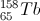undergoes an alpha decay?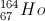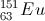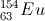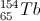Explanation:

Alpha decay is the loss of a helium atom from the original particle.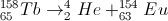The number of protons is reduced by two, and the nuclear mass is reduced by four.

### Example Question #2 : Radioactive Decay

Which type of nuclear decay is displayed below?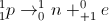Beta decay

Alpha decay

Gamma decay

Electron capture

Positron emission

Positron emission

Explanation:

Positron emission is when the nucleus ejects a positron, or positive equivalent of an electron. It is also known as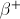decay.

Beta decay is when the nucleus ejects a beta particle (electron).

Alpha decay is when the nucleus ejects an alpha particle (helium nucleus).

Electron capture is when the nucleus caputres an electron from the inner most shell. This process converts a proton to a neutron.

Finally, gamma decay is when an excited nucleus emits gamma rays. Gamma rays have no mass or charge.

### Example Question #3 : Radioactive Decay

If an atom of potassium undergoes a positron emission, what will be the daughter nucleus?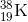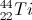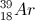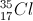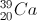Explanation:

An emission of a positron is also known to be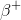decay. A positron emission can be viewed as a positron being ejected; therefore, when potassium undergoes a positron emission, the daughter nucleus will be argon (Ar) with the weight of 39.

To understand how the daughter nucleus was determined, create a positron emission reaction with potassium.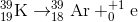### Example Question #4 : Radioactive Decay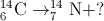Which of the following best describes the missing component in the reaction above?

An electron

An alpha particle

A neutron

A hydrogen nucleus

A photon

An electron

Explanation:

This is an example of beta decay because the atomic number increases (from 6 to 7). This means a neutron has decayed into a proton, which occurs by emitting an electron,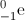.

### Example Question #5 : Radioactive Decay

The half-life of a particular isotope of radium is 1600 years. If a sample of this isotope originally has a mass of 120g, how long would it take the mass of this isotope to decrease to 15g?

4800 years

6400 years

3200 years

1600 years

800 years

4800 years

Explanation:

First note that 15g is 1/8 of the original 120-gram mass, so all but 1/8 of these radium nuclei have decayed. By definition, ½ of the nuclei decay during one half-life. So the number of nuclei remaining after n half-lives is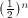. In this case, three half-lives have elapsed since,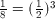. The answer is 3 * 1600 = 4800 years.

### Example Question #6 : Radioactive Decay

Thorium,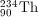, emits an alpha particle as it decays. Which of the following nuclei emerges from the decay?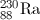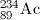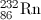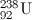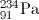Explanation:

In alpha decay a helium nucleus,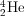, is emitted. Since the total number of nucleons must be conserved, the atomic number of the parent nucleus must decrease by two, and the mass number must decrease by four. Here, the new atomic number is 90 - 2 = 88, and new mass number is 234 - 4 = 230.

### Example Question #7 : Radioactive Decay

If gold,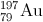, emits a beta particle, what will be the resulting product?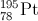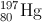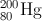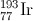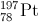Explanation:

When a nucleus ejects a beta particle, the element is undergoing beta decay. The ejection of a beta particle is equivalent to the ejection of an electron. When gold ejects an electron,, the product will be.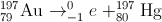### Example Question #8 : Radioactive Decay

In the operation of nuclear reactors, engineers make use of substances known as neutron poisons. These are used to help store nuclear waste and slow nuclear reactions, but are also generated naturally in nuclear chain reactions as a by-product. This natural by-product can stop the desirable chain reaction present in a nuclear reactor used for power generation.

For example, in nuclear power plants, U-235 is used as a fuel. U-235 absorbs a neutron, and subsequently generates neutrons (which power the chain reaction) and Xe-135. Xe-135 is a well-known neutron poison, and thus can impact the continued chain reaction of a nuclear power plant if it becomes over abundant during power generation.

To help account for this, engineers have developed measurements to quantify the impact of Xe-135 on nuclear operations. For instance, the time during which there is an inability to start a reactor due to the buildup of Xe-135 is referred to as the precluded start-up time. Also, the amount of time that the reactor cannot override the effects of built up Xe-135 is called poison outage time. Perhaps the most important measure that engineers have developed is the neutron absorption capacity (σ), which is measured in units of barns and is a function of microscopic cross section. Xe-135 has a neutron absorption capacity of 2.00 * 106 barns, while another common poison, Sm-149, has a neutron absorption capacity of 74,500 barns.

Nuclear reactions, such as the one described above with uranium, emit energy in the form of radiation. A scientist is considering three different forms of radiation to generate power. Which of the following types of radiation is highest in energy?

Electron capture

Positron emission

Explanation:

Gamma radiation is a highly energetic form of radiation, and is the type of radiation emitted when antimatter and matter annhilate each other upon contact.

### Example Question #9 : Radioactive Decay

In the operation of nuclear reactors, engineers make use of substances known as neutron poisons. These are used to help store nuclear waste and slow nuclear reactions, but are also generated naturally in nuclear chain reactions as a by-product. This natural by-product can stop the desirable chain reaction present in a nuclear reactor used for power generation.

For example, in nuclear power plants, U-235 is used as a fuel. U-235 absorbs a neutron, and subsequently generates neutrons (which power the chain reaction) and Xe-135. Xe-135 is a well-known neutron poison, and thus can impact the continued chain reaction of a nuclear power plant if it becomes over abundant during power generation.

To help account for this, engineers have developed measurements to quantify the impact of Xe-135 on nuclear operations. For instance, the time during which there is an inability to start a reactor due to the buildup of Xe-135 is referred to as the precluded start-up time. Also, the amount of time that the reactor cannot override the effects of built up Xe-135 is called poison outage time. Perhaps the most important measure that engineers have developed is the neutron absorption capacity (σ), which is measured in units of barns and is a function of microscopic cross section. Xe-135 has a neutron absorption capacity of 2.00 * 106 barns, while another common poison, Sm-149, has a neutron absorption capacity of 74,500 barns.

Xe-135 has a half life of around 9.2 hours. A reaction generated 70g of Xe-135, as measured by a scientist on a Friday afternoon. If he returned 46 hours later to study the sample, how much would remain?

3g

14g

4g

70g

2g

2g

Explanation:

The scientist allowed the substance to sit for a duration that was equal to five of its half life. The original sample decayed from 70g, to 35g, to 17.5g, to 8.75g, to 4.35g, to ~2 g.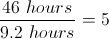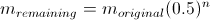n is equal to the number of half lives that have passed. For us, n is equal to 5.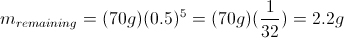### Example Question #10 : Radioactive Decay

In the operation of nuclear reactors, engineers make use of substances known as neutron poisons. These are used to help store nuclear waste and slow nuclear reactions, but are also generated naturally in nuclear chain reactions as a by-product. This natural by-product can stop the desirable chain reaction present in a nuclear reactor used for power generation.

For example, in nuclear power plants, U-235 is used as a fuel. U-235 absorbs a neutron, and subsequently generates neutrons (which power the chain reaction) and Xe-135. Xe-135 is a well-known neutron poison, and thus can impact the continued chain reaction of a nuclear power plant if it becomes over abundant during power generation.

To help account for this, engineers have developed measurements to quantify the impact of Xe-135 on nuclear operations. For instance, the time during which there is an inability to start a reactor due to the buildup of Xe-135 is referred to as the precluded start-up time. Also, the amount of time that the reactor cannot override the effects of built up Xe-135 is called poison outage time. Perhaps the most important measure that engineers have developed is the neutron absorption capacity (σ), which is measured in units of barns and is a function of microscopic cross section. Xe-135 has a neutron absorption capacity of 2.00 * 106 barns, while another common poison, Sm-149, has a neutron absorption capacity of 74,500 barns.

A scientist is studying a nuclear reaction similar to the one described in the passage. He realizes that the product atom has a mass number four less than the reactant, and an atomic number two less than the reactant. What is true of this type of radiation?

It results in the emission of either a helium nucleus or a heavy hydrogen nucleus, depending on conditions

It results in the emission of a heavy hydrogen nucleus, a type of ionizing radiation

It results in the emission of a helium nucleus, which is strictly a type of non-ionizing radiation

It results in the emission of a heavy hydrogen nucleus, which is strictly a type of non-ionizing radiation

It results in the emission of a helium nucleus, a type of ionizing radiation

This is an example of alpha radiation, which is the emission of a helium nucleus,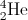, thus explaining the loss of two protons and four mass units from the original species. Because this is a nucleus, it can interact with other atoms in the environment to generate ionic species. This is in contrast to non-ionizing radiation, such as infrared or radio waves.